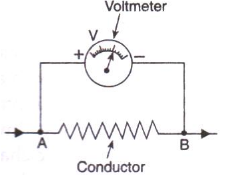# Solutions of Electricity (Page No- 6) - Physics By Lakhmir Singh, Class 10 Class 10 Notes | EduRev

## Class 10 : Solutions of Electricity (Page No- 6) - Physics By Lakhmir Singh, Class 10 Class 10 Notes | EduRev

The document Solutions of Electricity (Page No- 6) - Physics By Lakhmir Singh, Class 10 Class 10 Notes | EduRev is a part of the Class 10 Course Class 10 Physics Solutions By Lakhmir Singh & Manjit Kaur.
All you need of Class 10 at this link: Class 10

Page No:6

Question 16:
(a) What do you understand by the term “potential difference” ?
(b) What is meant by saying that the potential difference between two points is 1 volt ?
(c) What is the potential difference between the terminals of a battery if 250 joules of work is required to transfer 20 coulombs of charge from one terminal of battery to the other ?
(d) What is a voltmeter ? How is a voltmeter connected in the circuit to measure the potential difference between two points. Explain with the help of a diagram.
(e) State whether a voltmeter has a high resistance or a low resistance. Give reason for your answer.

Solution :
(a) Potential difference between two points in an electric circuit is defined as the amount of work done in moving a unit charge from one
point to the other point.
(b) The potential difference between two points is 1 volt means 1 joule of work is done in moving 1 coulomb of electric charge from one point to the other.
(c) Given: Work done = 250J, Charge moved = 20C.
we know that,Potential difference = Work done/Charge moved
= 250/20 = 12.5
(d) A voltmeter is a device which is used to measure the potential difference between two points in an electric circuit. Voltmeter is always connected in parallel across the two points where the potential difference is to be measured.(e) Voltmeter has a high resistance so that it takes a negligible current from the circuit.

Question 22:
Three 2 V cells are connected in series and used as a battery in a circuit.
(a) What is the p.d. at the terminals of the battery ?
(b) How many joules of electrical energy does 1 C gain on passing through (i) one cell (ii) all three cells ?

Solution :
(a) If three cells of 2 volt each are connected in series to make a battery, then the total potential difference between terminals of the battery will be 6V.
(b) (i) Given: p.d. = 2V, Charge moved = 1C
We know that
Work done = p.d. x charge moved
= 2 x 1
Work done = 2 joules
(ii) Given: p.d. = 6V, Charge moved = 1C
Work done = p.d. x charge moved
= 6 x1
Work done = 6 joule.

Question 23:
The atoms of copper contain electrons and the atoms of rubber also contain electrons. Then why does copper conduct electricity but rubber does not conduct electricity ?
Solution :
Copper has free electrons that are loosely held by the nuclei of the atoms. These free electrons result in conduction of electricity.
The electrons present in rubber are strongly held by the nuclei of its atoms. So, rubber does not have free electrons to conduct electricity.

Offer running on EduRev: Apply code STAYHOME200 to get INR 200 off on our premium plan EduRev Infinity!

94 docs

,

,

,

,

,

,

,

,

,

,

,

,

,

,

,

,

,

,

,

,

,

,

,

,

;